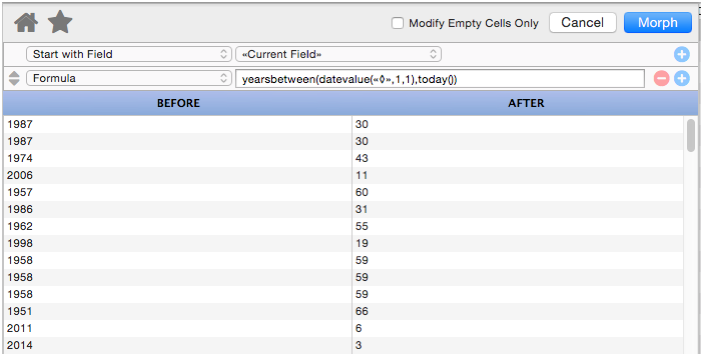When working with a numeric field, the Morph Field Dialog has about a dozen morph operations available for modifying numbers.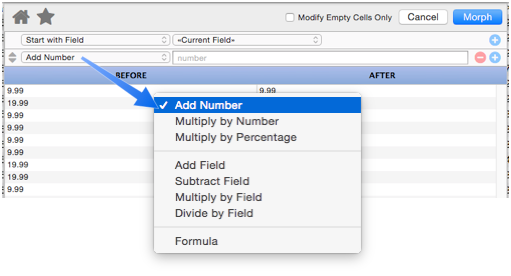The following sections discuss each of these morph operations.

This operation adds a fixed value to each cell. If you want to subtract, use a negative number.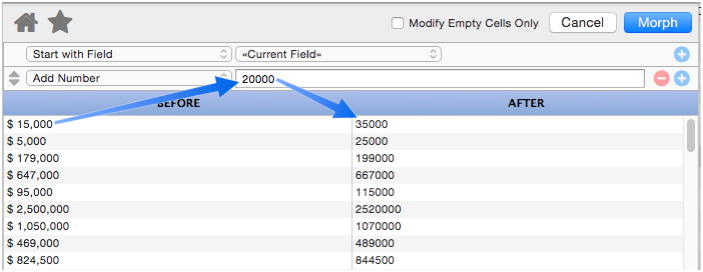#### Multiply by Number

This operation multiplies each number by a fixed amount.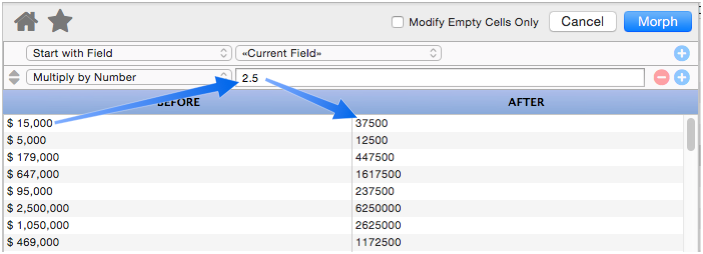#### Multiply by Percentage

This operation multiplies each number by a percentage.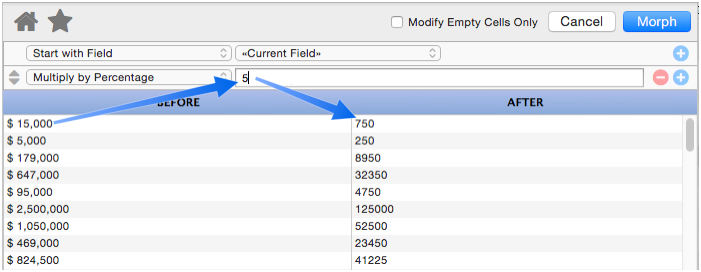This operation adds another numeric field to the data source. This example in an airport database calculates the altitude above sea level of each airport’s traffic pattern.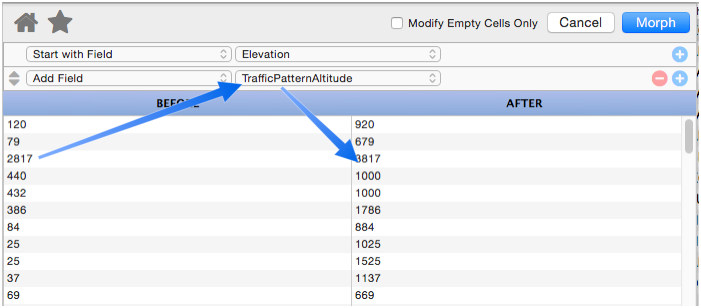#### Subtract Field

This operation subtracts another numeric field from the data source. This example from a real estate listing database calculates the price change from the original listing to the curent price.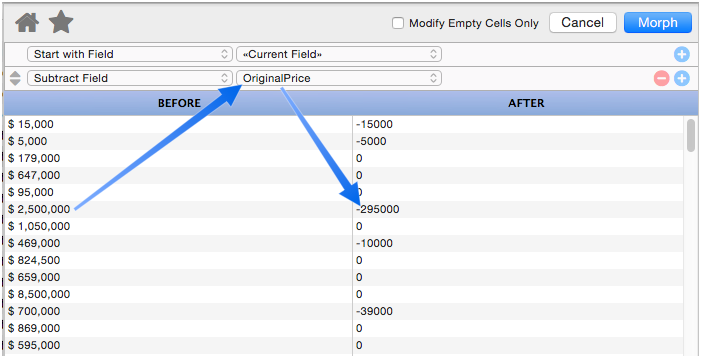#### Multiply by Field

This operation multiplies the data source by a numeric field.

#### Divide by Field

This operation divides the data source by a numeric field.

#### Formula

For more complex calculations you can use a formula. You can use a formula all on its own (see Morph Field Dialog) but you can also use a formula in combination with other manipulations. When used this way, the `«◊»` symbol will expand into the number produced by the previous manipulations. Then the result of the formula will be fed into any additional calculations below it.

This example calculates the age of a property in a real estate database, based on a field that contains the year the property was built.